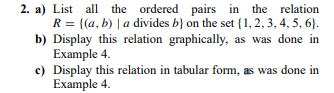# Question 2. a) List all the ordered pairs in the relation R = {(a, b) | a divides b) on the set (1, 2, 3, 4, 5, 6). b) Display this relation graphically, as was done in Example 4. c) Display this relation in tabular form, as was done in Example 4. 2. a) List all the ordered pairs in the relation R = {(a, b) | a divides b) on the set (1, 2, 3, 4, 5, 6). b) Display this relation graphically, as was done in Example 4. c) Display this relation in tabular form, as was done in Example 4.